# Contents

library(BiocStyle)

# 1 Introduction

The concept of mutational signatures was introduced in a series of papers by Ludmil Alexandrov et al. (Alexandrov, Nik-Zainal, Wedge, Aparicio, et al. 2013) and (Alexandrov, Nik-Zainal, Wedge, Campbell, et al. 2013). A computational framework was published (Alexandrov 2012) with the purpose to detect a limited number of mutational processes which then describe the whole set of SNVs (single nucleotide variants) in a cohort of cancer samples. The general approach (Alexandrov, Nik-Zainal, Wedge, Aparicio, et al. 2013) is as follows:

1. The SNVs are categorized by their nucleotide exchange. In total there are $$4 \times 3 = 12$$ different nucleotide exchanges, but if summing over reverse complements only $$12 / 2 = 6$$ different categories are left. For every SNV detected, the motif context around the position of the SNV is extracted. This may be a trinucleotide context if taking one base upstream and one base downstream of the position of the SNV, but larger motifs may be taken as well (e.g. pentamers). Taking into account the motif context increases combinatorial complexity: in the case of the trinucleotide context, there are $$4 \times 6 \times 4 = 96$$ different variant categories. These categories are called features in the following text. The number of features will be called $$n$$.
2. A cohort consists of different samples with the number of samples denoted by $$m$$. For each sample we can count the occurences of each feature, yielding an $$n$$-dimensional vector ($$n$$ being the number of features) per sample. For a cohort, we thus get an $$n \times m$$ -dimensional matrix, called the mutational catalogue $$V$$. It can be understood as a summary indicating which sample has how many variants of which category, but omitting the information of the genomic coordinates of the variants.
3. The mutational catalogue $$V$$ is quite big and still carries a lot of complexity. For many analyses a reduction of complexity is desirable. One way to achieve such a complexity reduction is a matrix decomposition: we would like to find two smaller matrices $$W$$ and $$H$$ which if multiplied would span a high fraction of the complexity of the big matrix $$V$$ (the mutational catalogue). Remember that $$V$$ is an $$n \times m$$ -dimensional matrix, $$n$$ being the number of features and $$m$$ being the number of samples. $$W$$ in this setting is an $$n \times l$$ -dimensional matrix and $$H$$ is an $$l \times m$$ -dimensional matrix. According to the nomeclature defined in (Alexandrov, Nik-Zainal, Wedge, Aparicio, et al. 2013), the columns of $$W$$ are called the mutational signatures and the columns of $$H$$ are called exposures. $$l$$ denotes the number of mutational signatures. Hence the signatures are $$n$$-dimensional vectors (with $$n$$ being the number of features), while the exposures are $$l$$-dimensional vectors ($$l$$ being the number of signatures). Note that as we are dealing with count data, we would like to have only positive entries in $$W$$ and $$H$$. A mathematical method which is able to do such a decomposition is the NMF (nonnegative matrix factorization). It basically solves the problem as illustrated in the following figure (Image taken from https://en.wikipedia.org/wiki/Non-negative_matrix_factorization):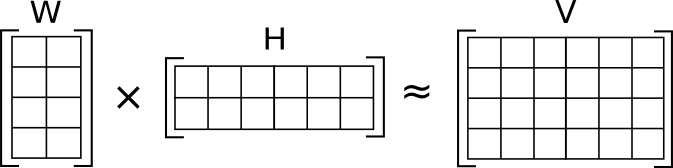Of course the whole concept only leads to a reduction in complexity if $$l < n$$, i.e. if the number of signatures is smaller than the number of features, as indicated in the above figure. Note that the NMF itself solves the above problem for a given number of signatures $$l$$. The framework of Ludmil Alexandrov et al. (Alexandrov, Nik-Zainal, Wedge, Aparicio, et al. 2013) performs not only the NMF decomposition itself, but also identifies the appropriate number of signatures by an iterative procedure.

Another software, the Bioconductor package SomaticSignatures to perform analyses of mutational signatures is available (Gehring et al. 2015) which allows the matrix decomposition to be performed by NMF and alternatively by PCA (principal component analysis). Both methods have in common that they can be used for discovery, i.e. for the extraction of new signatures. However, they only work well if the analyzed data set has a minimum size, i.e. a minimum number of samples and minimum numbers of counts per feature per sample.

The package YAPSA introduced here is complementary to these existing software packages. It is designed for a supervised analysis of mutational signatures, i.e. an analysis with already known signatures $$W$$, and with much lower requirements on statistical power of the input data.

# 2 The YAPSA package

In a context where mutational signatures $$W$$ are already known (because they were decribed and published as in (Alexandrov, Nik-Zainal, Wedge, Aparicio, et al. 2013) or they are available in a database as under http://cancer.sanger.ac.uk/cosmic/signatures), we might want to just find the exposures $$H$$ for these known signatures in the mutational catalogue $$V$$ of a given cohort. Mathematically, this is a different and potentially simpler task.

The YAPSA-package (Yet Another Package for Signature Analysis) presented here provides the function LCD (linear combination decomposition) to perform this task. The advantage of this method is that there are no constraints on the cohort size, so LCD can be run for as little as one sample and thus be used e.g. for signature analysis in personalized oncology. In contrast to NMF, LCD is very fast and requires very little computational resources. The YAPSA package provides additional functions for signature analysis, e.g. for stratifying the mutational catalogue to determine signature exposures in different strata, part of which will be discussed later in this vignette.

## 2.1 Linear Combination Decomposition (LCD)

In the following, we will denote the columns of $$V$$ by $$V_{(\cdot j)}$$, which corresponds to the mutational catalogue of sample $$j$$. Analogously we denote the columns of $$H$$ by $$H_{(\cdot j)}$$, which is the exposure vector of sample $$j$$. Then LCD is designed to solve the optimization problem:

1. \begin{aligned} \min_{H_{(\cdot j)} \in \mathbb{R}^l}||W \cdot H_{(\cdot j)} - V_{(\cdot j)}|| \quad \forall j \in \{1...m\} \\ \textrm{under the constraint of non-negativity:} \quad H_{(ij)} >= 0 \quad \forall i \in \{1...l\} \quad \forall j \in \{1...m\} \end{aligned}

Remember that $$j$$ is the index over samples, $$m$$ is the number of samples, $$i$$ is the index over signatures and $$l$$ is the number of signatures. LCD uses the function lsei from the package lsei (Wang, Lawson, and Hanson 2015). Note that the optimization procedure is carried out for every $$V_{(\cdot j)}$$, i.e. for every column of $$V$$ separately. Of course $$W$$ is constant, i.e. the same for every $$V_{(\cdot j)}$$.

This procedure is highly sensitive: as soon as a signature has a contribution or an exposure in at least one sample of a cohort, it will be reported (within the floating point precision of the operating system). This might blur the picture and counteracts the initial purpose of complexity reduction. Therefore there is a function LCD_complex_cutoff. This function takes as a second argument a cutoff (a value between zero and one). In the analysis, it will keep only those signatures which have a cumulative (over the cohort) normalized exposure greater than this cutoff. In fact it runs the LCD-procedure twice: once to find initial exposures, summing over the cohort and excluding the ones with too low a contribution as described just above, and a second time doing the analysis only with the signatures left over. Beside the exposures H corresponding to this reduced set of signatures, the function LCD_complex_cutoff also returns the reduced set of signatures itself.

Another R package for the supervised analysis of mutational signatures is available: deconstructSigs (Rosenthal et al. 2016). One difference between LCD_complex_cutoff as described here in YAPSA and the corresponding function whichSignatures in deconstructSigs is that LCD_complex_cutoff accepts different cutoffs and signature-specific cutoffs (accounting for potentially different detectability of different signatures), whereas in whichSignatures in deconstructSigs a general fixed cutoff is set to be 0.06. Furthermore, YAPSA has a function for a stratified analysis as described in the following paragraph.

## 2.2 Stratification of the Mutational Catalogue (SMC)

For some questions it is useful to assign the SNVs detected in the samples of a cohort to categories. In the following these categories have to be exclusive, i.e. one SNV must be in one and only one category. The categories will be called strata in the following and the procedure stratification. The number of strata will be denoted by $$s$$. For example one could evaluate whether an SNV falls into a region of high, intermediate or low mutation density by applying meaningful cutoffs on intermutation distance. Following the above convention, there are three strata: high, intermediate and low mutation density. If we have already performed an analysis of mutational signatures for the whole mutational catalogue of the cohort, we have identified a set of signatures of interest and the corresponding exposures. We now could ask the question if some of the signatures are enriched or depleted in one or the other stratum, yielding a strata-specific signature enrichment and depletion pattern. The function SMC (Stratification of the Mutational Catalogue) solves the stratified optimization problem:

1. \begin{aligned} \min_{H_{(\cdot j)}^k \in \mathbb{R}^l}||W \cdot H_{(\cdot j)}^{k} - V_{(\cdot j)}^{k}|| \quad \forall j,k \\ \textrm{under the constraint of non-negativity:} \quad H_{(ij)}^{k} >= 0 \quad \forall i,j,k \\ \textrm{and the additional contraint:} \quad \sum_{k=1}^{s} H^{k} = H \\ \textrm{where H is defined by the optimization:} \quad \min_{H_{(\cdot j)} \in \mathbb{R}^l}||W \cdot H_{(\cdot j)} - V_{(\cdot j)}|| \quad \forall j \\ \textrm{also under the constraint of non-negativity:} \quad H_{(ij)} >= 0 \quad \forall i,j \quad \textrm{and} \quad V = \sum_{k=1}^{s} V^{k} \end{aligned}

Remember that $$j$$ is the index over samples, $$m$$ is the number of samples, $$i$$ is the index over signatures, $$l$$ is the number of signatures, $$k$$ is the index over strata and $$s$$ is the number of strata. Note that the last two lines of equation (2) correspond to equation (1). The very last part of equation (2) reflects the additivity of the stratified mutational catalogues $$V^{k}$$ which is due to the fact that by definition the sets of SNVs they were constructed from (i.e. the strata) are exclusive.

The SMC-procedure can also be applied when an NMF analysis has been performed and the exposures $$\widetilde{H}$$ of this NMF analysis should be used as input and constraint for the SMC. It then solves the task:

1. \begin{aligned} \min_{H_{(\cdot j)}^k \in \mathbb{R}^l}||W \cdot H_{(\cdot j)}^{k} - V_{(\cdot j)}^{k}|| \quad \forall j,k \\ \textrm{under the constraint of non-negativity:} \quad H_{(ij)}^{k} >= 0 \quad \forall i,j,k \\ \textrm{and the additional contraint:} \quad \sum_{k=1}^{s} H^{k} = \widetilde{H} \\ \end{aligned}

Applying SMC that way, the initial LCD decomposition of the unstratified mutational catalogue is omitted and it’s result replaced by the exposures extracted by the NMF analysis.

# 3 Example: a cohort of B-cell lymphomas

We will now apply some functions of the YAPSA package to Whole Genome Sequencing datasets published in Alexandrov et al. (2013) First we have to load this data and get an overview (first subsection). Then we will load data on published signatures (second subsection). Only in the third subsection we will actually start using the YAPSA functions.

library(YAPSA)
library(knitr)
opts_chunk$set(echo=TRUE) opts_chunk$set(fig.show='asis')

## 3.1 Example data

In the following, we will load and get an overview of the data used in the analysis by Alexandrov et al. (Alexandrov, Nik-Zainal, Wedge, Aparicio, et al. 2013)

data("lymphoma_Nature2013_raw")

This created a data frame with 128639 rows. It is equivalent to executing the R code

lymphoma_Nature2013_ftp_path <- paste0(
"ftp://ftp.sanger.ac.uk/pub/cancer/AlexandrovEtAl/",
"somatic_mutation_data/Lymphoma B-cell/",
"Lymphoma B-cell_clean_somatic_mutations_",
"for_signature_analysis.txt")
header=FALSE,sep="\t")

The format is inspired by the vcf format with one line per called variant. Note that the files provided at that url have no header information, therefore we have to add some. We will also slightly adapt the data structure:

names(lymphoma_Nature2013_raw_df) <- c("PID","TYPE","CHROM","START",
"STOP","REF","ALT","FLAG")
lymphoma_Nature2013_df <- subset(lymphoma_Nature2013_raw_df,TYPE=="subs",
select=c(CHROM,START,REF,ALT,PID))
names(lymphoma_Nature2013_df) <- "POS"
kable(head(lymphoma_Nature2013_df))
CHROM POS REF ALT PID
1 183502381 G A 07-35482
18 60985506 T A 07-35482
18 60985748 G T 07-35482
18 60985799 T C 07-35482
2 242077457 A G 07-35482
6 13470412 C T 07-35482

Here, we have selected only the variants characterized as subs (those are the single nucleotide variants we are interested in for the mutational signatures analysis, small indels are filtered out by this step), so we are left with 128212 variants or rows. Note that there are 48 different samples:

unique(lymphoma_Nature2013_df$PID) ##  07-35482 1060 1061 1065 ##  1093 1096 1102 4101316 ##  4105105 4108101 4112512 4116738 ##  4119027 4121361 4125240 4133511 ##  4135350 4142267 4158726 4159170 ##  4163639 4175837 4177856 4182393 ##  4189200 4189998 4190495 4193278 ##  4194218 4194891 515 DLBCL-PatientA ##  DLBCL-PatientB DLBCL-PatientC DLBCL-PatientD DLBCL-PatientE ##  DLBCL-PatientF DLBCL-PatientG DLBCL-PatientH DLBCL-PatientI ##  DLBCL-PatientJ DLBCL-PatientK DLBCL-PatientL DLBCL-PatientM ##  EB2 FL009 FL-PatientA G1 ## 48 Levels: 07-35482 1060 1061 1065 1093 1096 1102 4101316 ... G1 For convenience later on, we annotate subgroup information to every variant (indirectly through the sample it occurs in). For reasons of simplicity, we also restrict the analysis to the Whole Genome Sequencing (WGS) datasets: lymphoma_Nature2013_df$SUBGROUP <- "unknown"
DLBCL_ind <- grep("^DLBCL.*",lymphoma_Nature2013_df$PID) lymphoma_Nature2013_df$SUBGROUP[DLBCL_ind] <- "DLBCL_other"
MMML_ind <- grep("^41[0-9]+$",lymphoma_Nature2013_df$PID)
lymphoma_Nature2013_df <- lymphoma_Nature2013_df[MMML_ind,]
data(lymphoma_PID)
for(my_PID in rownames(lymphoma_PID_df)) {
PID_ind <- which(as.character(lymphoma_Nature2013_df$PID)==my_PID) lymphoma_Nature2013_df$SUBGROUP[PID_ind] <-
lymphoma_PID_df$subgroup[which(rownames(lymphoma_PID_df)==my_PID)] } lymphoma_Nature2013_df$SUBGROUP <- factor(lymphoma_Nature2013_df$SUBGROUP) unique(lymphoma_Nature2013_df$SUBGROUP)
##  WGS_D WGS_F WGS_B WGS_I
## Levels: WGS_B WGS_D WGS_F WGS_I

### 3.1.2 Displaying example data

Rainfall plots provide a quick overview of the mutational load of a sample. To this end we have to compute the intermutational distances. But first we still do some reformatting…

lymphoma_Nature2013_df <- translate_to_hg19(lymphoma_Nature2013_df,"CHROM")
lymphoma_Nature2013_df$change <- attribute_nucleotide_exchanges(lymphoma_Nature2013_df) lymphoma_Nature2013_df <- lymphoma_Nature2013_df[order(lymphoma_Nature2013_df$PID,
lymphoma_Nature2013_df$CHROM, lymphoma_Nature2013_df$POS),]
lymphoma_Nature2013_df <- annotate_intermut_dist_cohort(lymphoma_Nature2013_df,
in_PID.field="PID")
data("exchange_colour_vector")
lymphoma_Nature2013_df$col <- exchange_colour_vector[lymphoma_Nature2013_df$change]

Now we can select one sample and make the rainfall plot. The plot function used here relies on the package gtrellis by Zuguang Gu (Zuguang Gu 2015).

choice_PID <- "4121361"
PID_df <- subset(lymphoma_Nature2013_df,PID==choice_PID)
trellis_rainfall_plot(PID_df,in_point_size=unit(0.5,"mm"))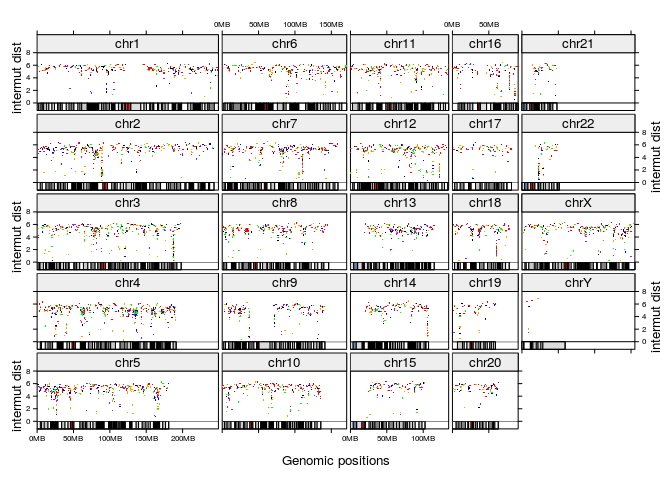This shows a rainfall plot typical for a lymphoma sample with clusters of increased mutation density e.g. at the immunoglobulin loci.

As stated above, one of the functions in the YAPSA package (LCD) is designed to do mutational signatures analysis with known signatures. There are (at least) two possible sources for signature data: i) the ones published initially by Alexandrov et al. (Alexandrov, Nik-Zainal, Wedge, Aparicio, et al. 2013), and ii) an updated and curated current set of mutational signatures is maintained by Ludmil Alexandrov at http://cancer.sanger.ac.uk/cosmic/signatures. The following three subsections describe how you can load the data from these resources. Alternatively, you can bypass the three following subsections because the signature datasets are also included in this package:

data(sigs)

However, the curated set of signatures might change in the future, therefore it is recommended to rebuild it from the above mentioned website as described in the following subsections.

We first load the (older) set of signatures as published in Alexandrov et al. (Alexandrov, Nik-Zainal, Wedge, Aparicio, et al. 2013):

Alex_signatures_path <- paste0("ftp://ftp.sanger.ac.uk/pub/cancer/",
"AlexandrovEtAl/signatures.txt")
kable(AlexInitialArtif_sig_df[c(1:9),c(1:4)])
Substitution.Type Trinucleotide Somatic.Mutation.Type Signature.1A
C>A ACA A[C>A]A 0.0112
C>A ACC A[C>A]C 0.0092
C>A ACG A[C>A]G 0.0015
C>A ACT A[C>A]T 0.0063
C>A CCA C[C>A]A 0.0067
C>A CCC C[C>A]C 0.0074
C>A CCG C[C>A]G 0.0009
C>A CCT C[C>A]T 0.0073
C>A GCA G[C>A]A 0.0083

We will now reformat the data frame:

Alex_rownames <- paste(AlexInitialArtif_sig_df[,1],
AlexInitialArtif_sig_df[,2],sep=" ")
select_ind <- grep("Signature",names(AlexInitialArtif_sig_df))
AlexInitialArtif_sig_df <- AlexInitialArtif_sig_df[,select_ind]
number_of_Alex_sigs <- dim(AlexInitialArtif_sig_df)
names(AlexInitialArtif_sig_df) <- gsub("Signature\\.","A",
names(AlexInitialArtif_sig_df))
rownames(AlexInitialArtif_sig_df) <- Alex_rownames
kable(AlexInitialArtif_sig_df[c(1:9),c(1:6)],
caption="Exemplary data from the initial Alexandrov signatures.")
Exemplary data from the initial Alexandrov signatures.
A1A A1B A2 A3 A4 A5
C>A ACA 0.0112 0.0104 0.0105 0.0240 0.0365 0.0149
C>A ACC 0.0092 0.0093 0.0061 0.0197 0.0309 0.0089
C>A ACG 0.0015 0.0016 0.0013 0.0019 0.0183 0.0022
C>A ACT 0.0063 0.0067 0.0037 0.0172 0.0243 0.0092
C>A CCA 0.0067 0.0090 0.0061 0.0194 0.0461 0.0097
C>A CCC 0.0074 0.0047 0.0012 0.0161 0.0614 0.0050
C>A CCG 0.0009 0.0013 0.0006 0.0018 0.0088 0.0028
C>A CCT 0.0073 0.0098 0.0011 0.0157 0.0432 0.0111
C>A GCA 0.0083 0.0169 0.0093 0.0107 0.0376 0.0119

This results in a data frame for signatures, containing 27 signatures as column vectors. It is worth noting that in the initial publication, only a subset of these 27 signatures were validated by an orthogonal sequencing technology. So we can filter down:

AlexInitialValid_sig_df <- AlexInitialArtif_sig_df[,grep("^A[0-9]+",
names(AlexInitialArtif_sig_df))]
number_of_Alex_validated_sigs <- dim(AlexInitialValid_sig_df)

We are left with 22 signatures.

An updated and curated set of mutational signatures is maintained by Ludmil Alexandrov at http://cancer.sanger.ac.uk/cosmic/signatures. We will use this set for the following analysis:

Alex_COSMIC_signatures_path <-
paste0("http://cancer.sanger.ac.uk/cancergenome/",
"assets/signatures_probabilities.txt")
Alex_COSMIC_rownames <- paste(AlexCosmicValid_sig_df[,1],
AlexCosmicValid_sig_df[,2],sep=" ")
COSMIC_select_ind <- grep("Signature",names(AlexCosmicValid_sig_df))
AlexCosmicValid_sig_df <- AlexCosmicValid_sig_df[,COSMIC_select_ind]
number_of_Alex_COSMIC_sigs <- dim(AlexCosmicValid_sig_df)
names(AlexCosmicValid_sig_df) <- gsub("Signature\\.","AC",
names(AlexCosmicValid_sig_df))
rownames(AlexCosmicValid_sig_df) <- Alex_COSMIC_rownames
kable(AlexCosmicValid_sig_df[c(1:9),c(1:6)],
caption="Exemplary data from the updated Alexandrov signatures.")
Exemplary data from the updated Alexandrov signatures.
AC1 AC2 AC3 AC4 AC5 AC6
C>A ACA 0.0110983 0.0006827 0.0221723 0.0365 0.0149415 0.0017
C>A ACC 0.0091493 0.0006191 0.0178717 0.0309 0.0089609 0.0028
C>A ACG 0.0014901 0.0000993 0.0021383 0.0183 0.0022078 0.0005
C>A ACT 0.0062339 0.0003239 0.0162651 0.0243 0.0092069 0.0019
C>G ACA 0.0018011 0.0002635 0.0240026 0.0097 0.0116710 0.0013
C>G ACC 0.0025809 0.0002699 0.0121603 0.0054 0.0072921 0.0012
C>G ACG 0.0005925 0.0002192 0.0052754 0.0031 0.0023038 0.0000
C>G ACT 0.0029640 0.0006110 0.0232777 0.0054 0.0116962 0.0018
C>T ACA 0.0295145 0.0074416 0.0178722 0.0120 0.0218392 0.0312

This results in a data frame containing 30 signatures as column vectors. For reasons of convenience and comparability with the initial signatures, we reorder the features. To this end, we adhere to the convention chosen in the initial publication by Alexandrov et al. (Alexandrov, Nik-Zainal, Wedge, Aparicio, et al. 2013) for the initial signatures.

COSMIC_order_ind <- match(Alex_rownames,Alex_COSMIC_rownames)
AlexCosmicValid_sig_df <- AlexCosmicValid_sig_df[COSMIC_order_ind,]
kable(AlexCosmicValid_sig_df[c(1:9),c(1:6)],
caption=paste0("Exemplary data from the updated Alexandrov ",
"signatures, rows reordered."))
Exemplary data from the updated Alexandrov signatures, rows reordered.
AC1 AC2 AC3 AC4 AC5 AC6
C>A ACA 0.0110983 0.0006827 0.0221723 0.0365 0.0149415 0.0017
C>A ACC 0.0091493 0.0006191 0.0178717 0.0309 0.0089609 0.0028
C>A ACG 0.0014901 0.0000993 0.0021383 0.0183 0.0022078 0.0005
C>A ACT 0.0062339 0.0003239 0.0162651 0.0243 0.0092069 0.0019
C>A CCA 0.0065959 0.0006774 0.0187817 0.0461 0.0096749 0.0101
C>A CCC 0.0073424 0.0002137 0.0157605 0.0614 0.0049523 0.0241
C>A CCG 0.0008928 0.0000068 0.0019634 0.0088 0.0028006 0.0091
C>A CCT 0.0071866 0.0004163 0.0147229 0.0432 0.0110135 0.0571
C>A GCA 0.0082326 0.0003520 0.0096965 0.0376 0.0118922 0.0024

Note that the order of the features, i.e. nucleotide exchanges in their trinucleotide content, is changed from the fifth line on as indicated by the row names.

### 3.2.3 Preparation for later analysis

For every set of signatures, the functions in the YAPSA package require an additional data frame containing meta information about the signatures. In that data frame you can specify the order in which the signatures are going to be plotted and the colours asserted to the different signatures. In the following subsection we will set up such a data frame. However, the respective data frames are also stored in the package. If loaded by data(sigs) the following code block can be bypassed.

signature_colour_vector <- c("darkgreen","green","pink","goldenrod",
"lightblue","blue","orangered","yellow",
"orange","brown","purple","red",
"darkblue","magenta","maroon","yellowgreen",
"violet","lightgreen","sienna4","deeppink",
"darkorchid","seagreen","grey10","grey30",
"grey50","grey70","grey90")
bio_process_vector <- c("spontaneous deamination","spontaneous deamination",
"APOBEC","BRCA1_2","Smoking","unknown",
"defect DNA MMR","UV light exposure","unknown",
"IG hypermutation","POL E mutations","temozolomide",
"unknown","APOBEC","unknown","unknown","unknown",
"unknown","unknown","unknown","unknown","unknown",
"nonvalidated","nonvalidated","nonvalidated",
"nonvalidated","nonvalidated")
AlexInitialArtif_sigInd_df <- data.frame(sig=colnames(AlexInitialArtif_sig_df))
AlexInitialArtif_sigInd_df$index <- seq_len(dim(AlexInitialArtif_sigInd_df)) AlexInitialArtif_sigInd_df$colour <- signature_colour_vector
AlexInitialArtif_sigInd_df$process <- bio_process_vector COSMIC_signature_colour_vector <- c("green","pink","goldenrod", "lightblue","blue","orangered","yellow", "orange","brown","purple","red", "darkblue","magenta","maroon", "yellowgreen","violet","lightgreen", "sienna4","deeppink","darkorchid", "seagreen","grey","darkgrey", "black","yellow4","coral2","chocolate2", "navyblue","plum","springgreen") COSMIC_bio_process_vector <- c("spontaneous deamination","APOBEC", "defect DNA DSB repair hom. recomb.", "tobacco mutatgens, benzo(a)pyrene", "unknown", "defect DNA MMR, found in MSI tumors", "UV light exposure","unknown","POL eta and SHM", "altered POL E", "alkylating agents, temozolomide", "unknown","APOBEC","unknown", "defect DNA MMR","unknown","unknown", "unknown","unknown", "associated w. small indels at repeats", "unknown","aristocholic acid","unknown", "aflatoxin","unknown","defect DNA MMR", "unknown","unknown","tobacco chewing","unknown") AlexCosmicValid_sigInd_df <- data.frame(sig=colnames(AlexCosmicValid_sig_df)) AlexCosmicValid_sigInd_df$index <- seq_len(dim(AlexCosmicValid_sigInd_df))
AlexCosmicValid_sigInd_df$colour <- COSMIC_signature_colour_vector AlexCosmicValid_sigInd_df$process <- COSMIC_bio_process_vector

## 3.3 Performing an LCD analysis

Now we can start using the functions from the YAPSA package. We will start with a mutational signatures analysis using known signatures (the ones we loaded in the above paragraph). For this, we will use the functions LCD and LCD_complex_cutoff.

### 3.3.1 Building a mutational catalogue

This section uses functions which are to a large extent wrappers for functions in the package SomaticSignatures by Julian Gehring (Gehring et al. 2015).

library(BSgenome.Hsapiens.UCSC.hg19)
word_length <- 3

lymphomaNature2013_mutCat_list <-
create_mutation_catalogue_from_df(
lymphoma_Nature2013_df,
this_seqnames.field = "CHROM", this_start.field = "POS",
this_end.field = "POS", this_PID.field = "PID",
this_subgroup.field = "SUBGROUP",
this_refGenome = BSgenome.Hsapiens.UCSC.hg19,
this_wordLength = word_length)

The function create_mutation_catalogue_from_df returns a list object with several entries. We will use the one called matrix.

names(lymphomaNature2013_mutCat_list)
##  "matrix" "frame"
lymphomaNature2013_mutCat_df <- as.data.frame(
lymphomaNature2013_mutCat_list$matrix) kable(lymphomaNature2013_mutCat_df[c(1:9),c(5:10)]) 4116738 4119027 4121361 4125240 4133511 4135350 C>A ACA 127 31 72 34 49 75 C>A ACC 104 36 39 19 36 80 C>A ACG 13 2 2 1 6 8 C>A ACT 102 33 48 22 47 56 C>A CCA 139 43 47 29 51 70 C>A CCC 66 34 35 7 25 42 C>A CCG 9 7 6 3 7 11 C>A CCT 167 47 50 32 58 84 C>A GCA 90 47 66 29 45 66 ### 3.3.2 LCD analysis without any cutoff The LCD function performs the decomposition of a mutational catalogue into a priori known signatures and the respective exposures to these signatures as described in the second section of this vignette. We use the “new” signatures from the COSMIC website. current_sig_df <- AlexCosmicValid_sig_df current_sigInd_df <- AlexCosmicValid_sigInd_df lymphomaNature2013_COSMICExposures_df <- LCD(lymphomaNature2013_mutCat_df,current_sig_df) Some adaptation (extracting and reformatting the information which sample belongs to which subgroup): COSMIC_subgroups_df <- make_subgroups_df(lymphoma_Nature2013_df, lymphomaNature2013_COSMICExposures_df) The resulting signature exposures can be plotted using custom plotting functions. First as absolute exposures: exposures_barplot( in_exposures_df = lymphomaNature2013_COSMICExposures_df, in_subgroups_df = COSMIC_subgroups_df)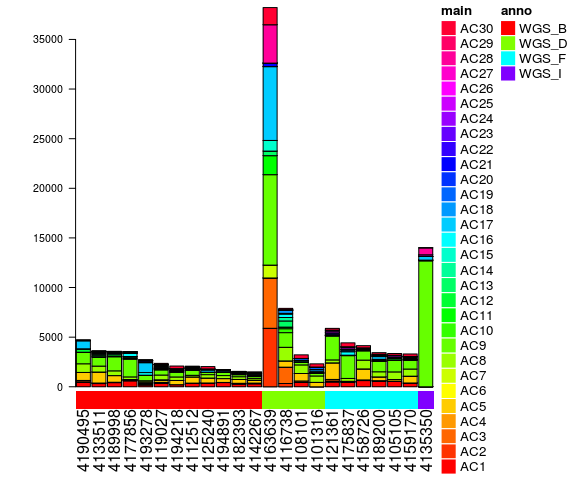Here, as no colour information was given to the plotting function exposures_barplot, the identified signatures are coloured in a rainbow palette. If you want to assign colours to the signatures, this is possible via a data structure of type sigInd_df. exposures_barplot( in_exposures_df = lymphomaNature2013_COSMICExposures_df, in_signatures_ind_df = current_sigInd_df, in_subgroups_df = COSMIC_subgroups_df)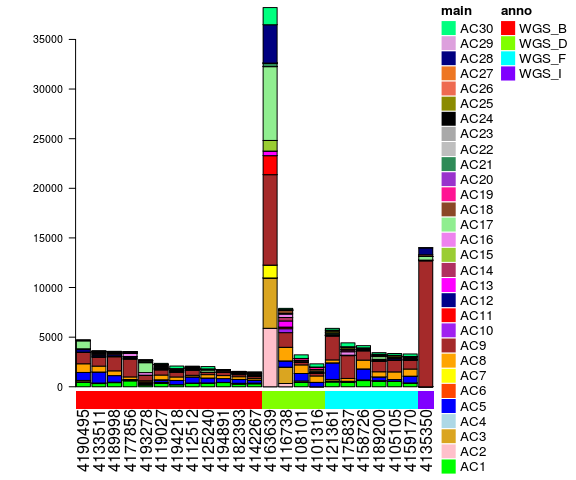This figure has a colour coding which suits our needs, but there is one slight inconsistency: colour codes are assigned to all 30 provided signatures, even though some of them might not have any contributions in this cohort: rowSums(lymphomaNature2013_COSMICExposures_df) ## AC1 AC2 AC3 AC4 AC5 AC6 ## 7600.27525 6876.09167 7532.32331 0.00000 11400.54366 165.58927 ## AC7 AC8 AC9 AC10 AC11 AC12 ## 1360.82415 10792.42959 40780.48663 750.23833 2330.47326 1416.83092 ## AC13 AC14 AC15 AC16 AC17 AC18 ## 1278.21698 972.57326 1277.88700 1616.06653 10715.25678 1345.94160 ## AC19 AC20 AC21 AC22 AC23 AC24 ## 1269.85447 231.99906 909.70507 48.66667 61.22117 0.00000 ## AC25 AC26 AC27 AC28 AC29 AC30 ## 639.24910 258.01427 382.52350 4768.12968 76.81338 4745.15419 This can be overcome by using LCD_complex_cutoff. Is requires an additional parameter: in_cutoff_vector; this is already the more general framework which will be explained in more detail in the following section. zero_cutoff_vector <- rep(0,dim(current_sig_df)) CosmicValid_cutoffZero_LCDlist <- LCD_complex_cutoff( in_mutation_catalogue_df = lymphomaNature2013_mutCat_df, in_signatures_df = current_sig_df, in_cutoff_vector = zero_cutoff_vector, in_sig_ind_df = current_sigInd_df) We can re-create the subgroup information (even though this is identical to the already determined one): COSMIC_subgroups_df <- make_subgroups_df(lymphoma_Nature2013_df, CosmicValid_cutoffZero_LCDlist$exposures)

And if we plot this, we obtain:

exposures_barplot(
in_exposures_df = CosmicValid_cutoffZero_LCDlist$exposures, in_signatures_ind_df = CosmicValid_cutoffZero_LCDlist$out_sig_ind_df,
in_subgroups_df = COSMIC_subgroups_df)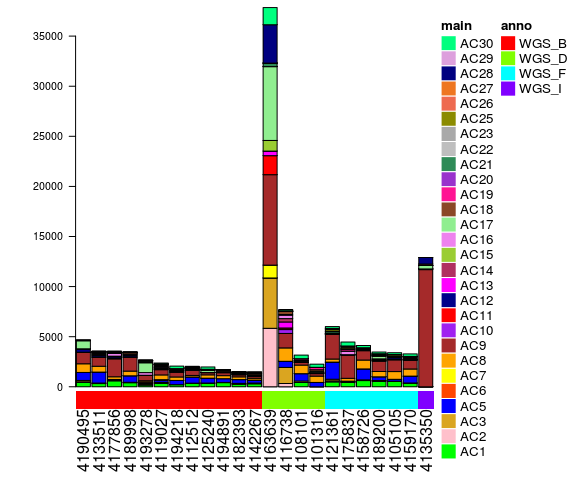Note that this time, only the 28 signatures which actually have a contribution to this cohort are displayed in the legend.

Of course, also relative exposures may be plotted:

exposures_barplot(
in_exposures_df = CosmicValid_cutoffZero_LCDlist$norm_exposures, in_signatures_ind_df = CosmicValid_cutoffZero_LCDlist$out_sig_ind_df,
in_subgroups_df = COSMIC_subgroups_df)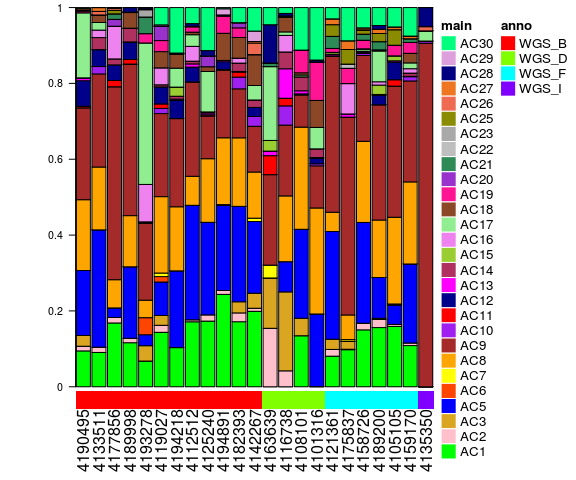### 3.3.3 LCD analysis with a generalized cutoff

Now let’s rerun the analysis with a cutoff to discard signatures with insufficient cohort-wide contribution.

my_cutoff <- 0.06

The cutoff of 0.06 means that a signature is kept if it’s exposure represents at least 6% of all SNVs in the cohort. We will use the function LCD_complex_cutoff instead of LCD.

general_cutoff_vector <- rep(my_cutoff,dim(current_sig_df))
CosmicValid_cutoffGen_LCDlist <- LCD_complex_cutoff(
in_mutation_catalogue_df = lymphomaNature2013_mutCat_df,
in_signatures_df = current_sig_df,
in_cutoff_vector = general_cutoff_vector,
in_sig_ind_df = current_sigInd_df)

At the chosen cutoff of 0.06, we are left with 6 signatures. We can look at these signatures in detail and their attributed biological processes:

kable(CosmicValid_cutoffGen_LCDlist$out_sig_ind_df, row.names=FALSE, caption=paste0("Signatures with cohort-wide exposures > ",my_cutoff)) Signatures with cohort-wide exposures > 0.06 sig index colour process AC1 1 green spontaneous deamination AC3 3 goldenrod defect DNA DSB repair hom. recomb. AC5 5 blue unknown AC8 8 orange unknown AC9 9 brown POL eta and SHM AC17 17 lightgreen unknown Again we can plot absolute exposures: exposures_barplot( in_exposures_df = CosmicValid_cutoffGen_LCDlist$exposures,
in_signatures_ind_df = CosmicValid_cutoffGen_LCDlist$out_sig_ind_df, in_subgroups_df = COSMIC_subgroups_df)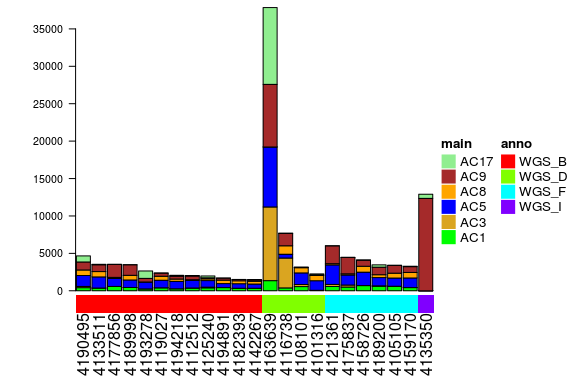And relative exposures: exposures_barplot( in_exposures_df = CosmicValid_cutoffGen_LCDlist$norm_exposures,
in_signatures_ind_df = CosmicValid_cutoffGen_LCDlist$out_sig_ind_df, in_subgroups_df = COSMIC_subgroups_df)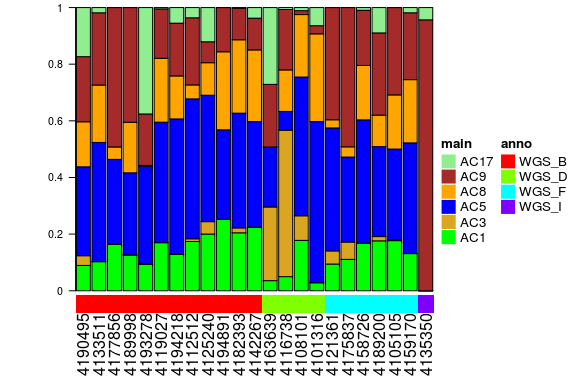### 3.3.4 LCD analysis with signature-specific cutoffs When using LCD_complex_cutoff, we have to supply a vector of cutoffs with as many entries as there are signatures. In the analysis carried out above, these were all equal, but this is not a necessary condition. Indeed it may make sense to provide different cutoffs for different signatures. specific_cutoff_vector <- general_cutoff_vector specific_cutoff_vector[c(1,5)] <- 0 specific_cutoff_vector ##  0.00 0.06 0.06 0.06 0.00 0.06 0.06 0.06 0.06 0.06 0.06 0.06 0.06 0.06 ##  0.06 0.06 0.06 0.06 0.06 0.06 0.06 0.06 0.06 0.06 0.06 0.06 0.06 0.06 ##  0.06 0.06 In this example, the cutoff for signatures AC1 and AC5 is thus set to 0, whereas the cutoffs for all other signatures remains at 0.06. Running the function LCD_complex_cutoff is completely analogous: CosmicValid_cutoffSpec_LCDlist <- LCD_complex_cutoff( in_mutation_catalogue_df = lymphomaNature2013_mutCat_df, in_signatures_df = current_sig_df, in_cutoff_vector = specific_cutoff_vector, in_sig_ind_df = current_sigInd_df) Plotting absolute exposures for visualization: exposures_barplot( in_exposures_df = CosmicValid_cutoffSpec_LCDlist$exposures,
in_signatures_ind_df = CosmicValid_cutoffSpec_LCDlist$out_sig_ind_df, in_subgroups_df = COSMIC_subgroups_df)And relative exposures: exposures_barplot( in_exposures_df = CosmicValid_cutoffSpec_LCDlist$norm_exposures,
in_signatures_ind_df = CosmicValid_cutoffSpec_LCDlist$out_sig_ind_df, in_subgroups_df = COSMIC_subgroups_df)Note that the signatures extracted with the signature-specific cutoffs are the same in the example displayed here. Depending on the analyzed cohort and the choice of cutoffs, the extracted signatures may vary considerably. ## 3.4 Cluster samples based on their signature exposures To identify groups of samples which were exposed to similar mutational processes, the exposure vectors of the samples can be compared. The YAPSA package provides a custom function for this task: complex_heatmap_exposures, which uses the package ComplexHeatmap by Zuguang Gu (Z Gu 2015). It produces output as follows: complex_heatmap_exposures(CosmicValid_cutoffGen_LCDlist$norm_exposures,
COSMIC_subgroups_df,
CosmicValid_cutoffGen_LCDlist$out_sig_ind_df, in_data_type="norm exposures", in_subgroup_colour_column="col", in_method="manhattan", in_subgroup_column="subgroup")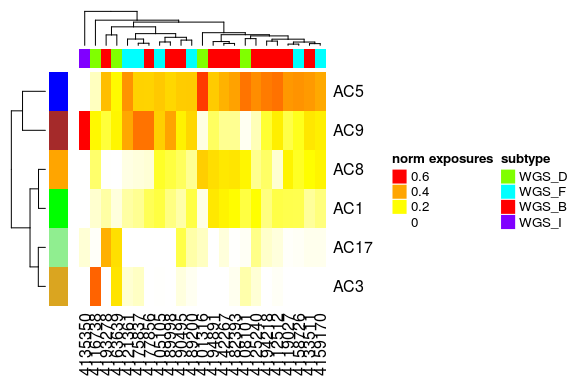If you are interested only in the clustering and not in the heatmap information, you could also use hclust_exposures: hclust_list <- hclust_exposures(CosmicValid_cutoffGen_LCDlist$norm_exposures,
COSMIC_subgroups_df,
in_method="manhattan",
in_subgroup_column="subgroup")

The dendrogram produced by either the function complex_heatmap_exposures or the function hclust_exposures can be cut to yield signature exposure specific subgroups of the PIDs.

cluster_vector <- cutree(hclust_list$hclust,k=4) COSMIC_subgroups_df$cluster <- cluster_vector
subgroup_colour_vector <- rainbow(length(unique(COSMIC_subgroups_df$cluster))) COSMIC_subgroups_df$cluster_col <-
subgroup_colour_vector[factor(COSMIC_subgroups_df$cluster)] complex_heatmap_exposures(CosmicValid_cutoffGen_LCDlist$norm_exposures,
COSMIC_subgroups_df,
CosmicValid_cutoffGen_LCDlist$out_sig_ind_df, in_data_type="norm exposures", in_subgroup_colour_column="cluster_col", in_method="manhattan", in_subgroup_column="cluster")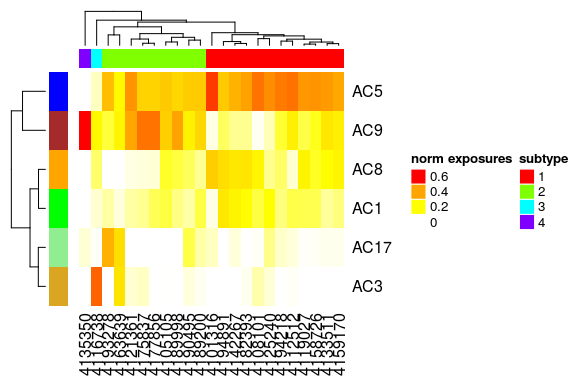## 3.5 Performing a stratification based on mutation density We will now use the intermutational distances computed above. We set cutoffs for the intermutational distance at 1000 and 100000 bp, leading to three strata. We annotate to every variant in which stratum it falls. lymphoma_Nature2013_df$density_cat <- cut(lymphoma_Nature2013_df$dist, c(0,1001,100001,Inf), right=FALSE, labels=c("high","intermediate", "background")) The following table shows the distribution of variants over strata: temp_df <- data.frame(table(lymphoma_Nature2013_df$density_cat))
names(temp_df) <- c("Stratum","Cohort-wide counts")
kable(temp_df, caption=paste0("Strata for the SMC of mutation density"))
Strata for the SMC of mutation density
Stratum Cohort-wide counts
high 6818
intermediate 62131
background 50675

We now have everything at hand to carry out a stratified signature analysis:

strata_order_ind <- c(1,3,2)
mut_density_list <- run_SMC(lymphoma_Nature2013_df,
CosmicValid_cutoffGen_LCDlist$signatures, CosmicValid_cutoffGen_LCDlist$out_sig_ind_df,
COSMIC_subgroups_df,
column_name="density_cat",
refGenome=BSgenome.Hsapiens.UCSC.hg19,
cohort_method_flag="norm_PIDs",
in_strata_order_ind=strata_order_ind)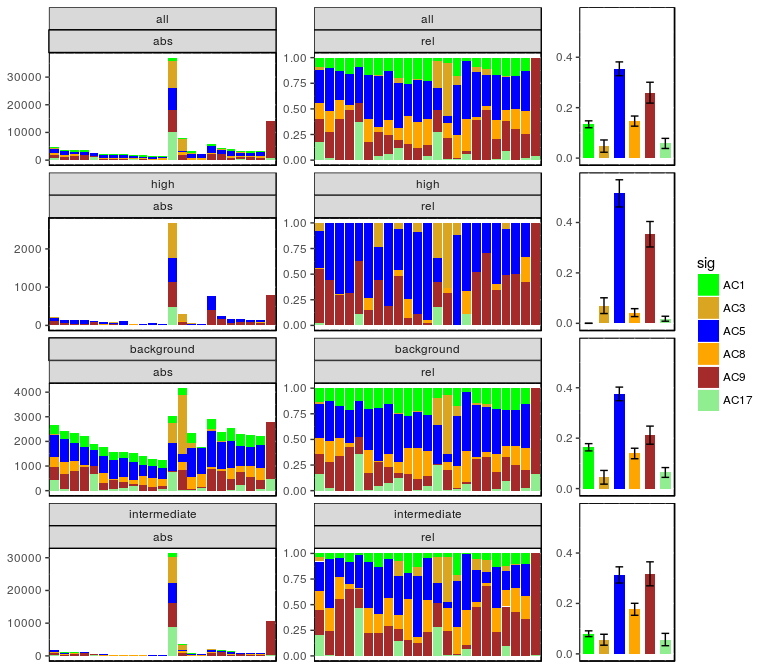This produces a multi-panel figure with 4 rows of plots. The first row visualizes the signature distribution over the whole cohort without stratification, followed by one row of plots per stratum. Hence in our example we have four rows of graphs with three (exclusive) strata as input. Each row consists of three plots. The left plots show absolute exposures in the respective stratum as stacked barplots on a per sample basis. The middle plots show relative exposures in the respective stratum on a per sample basis as stacked barplots. The right plots shows cohort-wide averages of the relative exposures in the respective stratum. The error bars indicate the standard error of the mean (SEM).

To test for statistical significance of potential differences in the signature exposures (i.e. signature enrichment and depletion patterns) between the different strata, we can use the Kruskal-Wallis test, as the data is grouped into (potentially more than two) categories and might not follow a normal distribution. As we are testing the different signatures on the same stratification, we have to correct for multiple testing. In order to control the false discovery rate (FDR), the Benjamini-Hochberg correction is appropriate.

stat_mut_density_list <- stat_test_SMC(mut_density_list,in_flag="norm")
kable(stat_mut_density_list$kruskal_df, caption=paste0("Results of Kruskal tests for cohort-wide exposures over", " strata per signature without and with correction for ", "multiple testing.")) Results of Kruskal tests for cohort-wide exposures over strata per signature without and with correction for multiple testing. Kruskal_statistic df Kruskal_p_val Kruskal_p_val_BH AC1 49.9620038 2 0.0000000 0.0000000 AC3 0.6985189 2 0.7052101 0.7052101 AC5 10.2070496 2 0.0060753 0.0091129 AC8 19.4325695 2 0.0000603 0.0001809 AC9 5.8243570 2 0.0543572 0.0652286 AC17 10.4314799 2 0.0054304 0.0091129 In the following paragraph we perform post-hoc tests for those signatures where the Kruskal-Wallis test, as evaluated above, has given a significant result. significance_level <- 0.05 for(i in seq_len(dim(stat_mut_density_list$kruskal_df))){
if(stat_mut_density_list$kruskal_df$Kruskal_p_val_BH[i]<significance_level){
print(paste0("Signature: ",rownames(stat_mut_density_list$kruskal_df)[i])) print(stat_mut_density_list$kruskal_posthoc_list[[i]])
}
}
##  "Signature: AC1"
##
##  Pairwise comparisons using Tukey and Kramer (Nemenyi) test
##                    with Tukey-Dist approximation for independent samples
##
## data:  sig_exposures_vector and sig_strata_vector
##
##              background high
## high         2.3e-11    -
## intermediate 0.028      5.3e-05
##
## P value adjustment method: none
##  "Signature: AC5"
##
##  Pairwise comparisons using Tukey and Kramer (Nemenyi) test
##                    with Tukey-Dist approximation for independent samples
##
## data:  sig_exposures_vector and sig_strata_vector
##
##              background high
## high         0.1706     -
## intermediate 0.3466     0.0041
##
## P value adjustment method: none
##  "Signature: AC8"
##
##  Pairwise comparisons using Tukey and Kramer (Nemenyi) test
##                    with Tukey-Dist approximation for independent samples
##
## data:  sig_exposures_vector and sig_strata_vector
##
##              background high
## high         0.0051     -
## intermediate 0.5240     7.7e-05
##
## P value adjustment method: none
##  "Signature: AC17"
##
##  Pairwise comparisons using Tukey and Kramer (Nemenyi) test
##                    with Tukey-Dist approximation for independent samples
##
## data:  sig_exposures_vector and sig_strata_vector
##
##              background high
## high         0.0078     -
## intermediate 0.3201     0.2674
##
## P value adjustment method: none

From this analysis, we can see that a distinct signature enrichment and depletion pattern emerges:

1. Stratum of high mutation density: Enrichment of signatures AC5 (significant) and AC9, depletion of signatures AC1 (significant), AC2, AC8 (significant) and AC17
2. Background: signature distribution very similar to the one of the complete mutational catalogue (first row)
3. Stratum of intermediate mutation density: intermediate signature enrichment and depletion pattern between the strata of high mutation density and background.